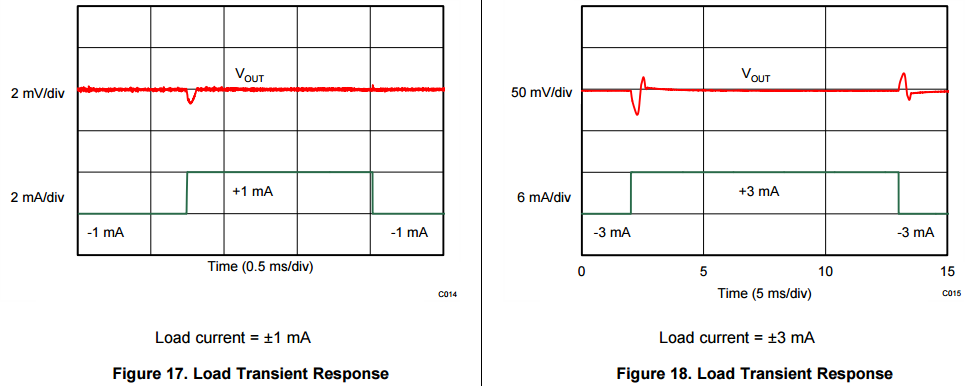If you have a related question, please click the "Ask a related question" button in the top right corner. The newly created question will be automatically linked to this question.

# REF6150: About REF6150 output impedance

Part Number: REF6150
Other Parts Discussed in Thread: REF6125

Dear,

I am designing a circuit to convert single end signal(Vp2p:5V Bias:2.5V) to differential signal(Vp2p:2V, Bias 1V).

And the SNR requirement is 78 db.

So I need a 2.5v reference voltage with a very low output impedance(<100m ohm). I want to choose REF6150 as 2.5v reference voltage.

Here are the picture I pick up from the datasheet of REF6150

The design simulation say the output impedance is less than 25m ohm.(That is very low value)

But the test result show the output impedance is about 2 ohm(25mV ripple/9mA transient current) in figure 18.Why is there the output impedance difference between the figure 16 and figure 18?

• Hi,

I will do some digging into the graphs/output impedance and I will let you know what I find.

Also, the REF6150 is 5V reference. The REF6125 is the 2.5V version.

Regards, Diego Lewis

• Hi,

The output impedance is a small signal parameter. That value is supported for output current changes in the range of a few hundred uA.

Figures 17 and 18 show how the driver loop responds to DC load switching. This behavior depends on other factors besides output impedance.

Regards, Diego Lewis